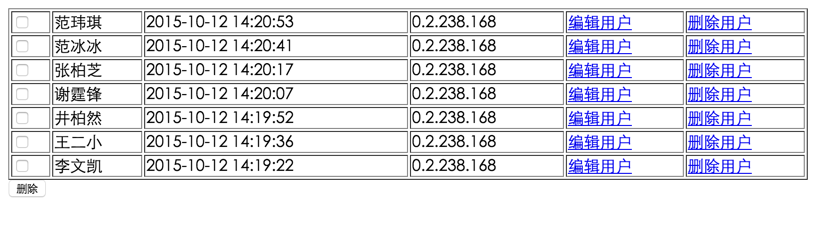• 注册

# php数据库操作之通过步骤做一个列表显示

```\$conn = mysqli_connect('localhost', 'root', 'secret', 'book');
if (mysqli_errno(\$conn)) {
mysqli_error(\$conn);
exit;
}
mysqli_set_charset(\$conn, 'utf8');```

```\$sql = "select id,username,createtime,createip from user order by id desc";
\$result = mysqli_query(\$conn, \$sql);```

```if(\$result && mysqli_num_rows(\$result)){
//显示列表代码段
}else{
//提示没有结果的代码段
}```

```  echo '<table width="800" border="1">';
while (\$row = mysqli_fetch_assoc(\$result)) {
echo '<tr>';
echo '<td>' . \$row['username'] . '</td>';
echo '<td>' . date('Y-m-d H:i:s', \$row['createtime']) . '</td>';
echo '<td>' . long2ip(\$row['createip']) . '</td>';
echo '</tr>';
}
echo '</table>';```

1.在删除的时候我们分为单选删除和多选删除。

2.而编辑的时候，我们会选择一个用户1.单选择删除和编辑时需使用get方法传入ID，我们才知道要编辑或者删除的是哪个用户。

2.多选删除时，需要使用传入多个用户。因此，我们可以使用form表单，使用post方法来提交这批用户ID。

`echo '<td><a href="delete.php?id=' . \$row['id'] . '">删除用户</a></td>';`

编辑用户也是同理，我们在edit.php加上?写上id和值，点击时就知道是需要编辑的哪个用户了。

`echo '<td><a href="edit.php?id=' . \$row['id'] . '">编辑用户</a></td>';`

```<form action="delete.php" method="post">
echo '<td><input type="checkbox" name="id[]" value="' . \$row['id'] . '" /></td>';
echo '<input type="submit" value="删除" />';
echo '</form>';```

```echo '<form action="delete.php" method="post">';
echo '<table width="800" border="1">';
while (\$row = mysqli_fetch_assoc(\$result)) {
echo '<tr>';
echo '<td><input type="checkbox" name="id[]" value="' . \$row['id'] . '" /></td>';
echo '<td>' . \$row['username'] . '</td>';
echo '<td>' . date('Y-m-d H:i:s', \$row['createtime']) . '</td>';
echo '<td>' . long2ip(\$row['createip']) . '</td>';
echo '<td><a href="edit.php?id=' . \$row['id'] . '">编辑用户</a></td>';
echo '<td><a href="delete.php?id=' . \$row['id'] . '">删除用户</a></td>';
echo '</tr>';
}
echo '</table>';
echo '<input type="submit" value="删除" />';
echo '</form>';```

`mysqli_close(\$conn);`

```<?php

\$conn = mysqli_connect('localhost', 'root', 'secret', 'book');

if (mysqli_errno(\$conn)) {
mysqli_error(\$conn);
exit;
}

mysqli_set_charset(\$conn, 'utf8');

\$sql = "select id,username,createtime,createip from user order by id desc";

\$result = mysqli_query(\$conn, \$sql);

if (\$result && mysqli_num_rows(\$result)) {

echo '<table width="800" border="1">';

while (\$row = mysqli_fetch_assoc(\$result)) {

echo '<tr>';

echo '<td>' . \$row['username'] . '</td>';
echo '<td>' . date('Y-m-d H:i:s', \$row['createtime']) . '</td>';
echo '<td>' . long2ip(\$row['createip']) . '</td>';
echo '<td><a href="edit.php?id=' . \$row['id'] . '">编辑用户</a></td>';
echo '<td><a href="delete.php?id=' . \$row['id'] . '">删除用户</a></td>';

echo '</tr>';
}

echo '</table>';

} else {
echo '没有数据';
}

mysqli_close(\$conn);
?>```

||
<?php \$conn = mysqli_connect('localhost', 'root', 'secret', 'book'); if (mysqli_errno(\$conn)) { mysqli_error(\$conn); exit; } mysqli_set_charset(\$conn, 'utf8'); \$sql = "select id,username,createtime,createip from user order by id desc"; \$result = mysqli_query(\$conn, \$sql); if (\$result && mysqli_num_rows(\$result)) { echo '<table width="800" border="1">'; while (\$row = mysqli_fetch_assoc(\$result)) { echo '<tr>'; echo '<td>' . \$row['username'] . '</td>'; echo '<td>' . date('Y-m-d H:i:s', \$row['createtime']) . '</td>'; echo '<td>' . long2ip(\$row['createip']) . '</td>'; echo '<td><a href="edit.php?id=' . \$row['id'] . '">编辑用户</a></td>'; echo '<td><a href="delete.php?id=' . \$row['id'] . '">删除用户</a></td>'; echo '</tr>'; } echo '</table>'; } else { echo '没有数据'; } mysqli_close(\$conn); ?>